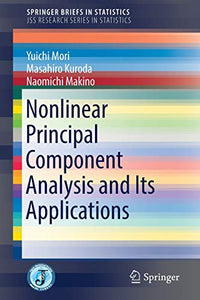# Nonlinear Principal Component Analysis and Its Applications

Yuichi Mori

Format: Print Book

ISBN: 9789811001574

• SGD 85.79
Unit price per
• Save SGD 9.53
Tax included.

Will not ship until

This book expounds the principle and related applications of nonlinear principal component analysis (PCA), which is useful method to analyze mixed measurement levels data.
In the part dealing with the principle, after a brief introduction of ordinary PCA, a PCA for categorical data (nominal and ordinal) is introduced as nonlinear PCA, in which an optimal scaling technique is used to quantify the categorical variables. The alternating least squares (ALS) is the main algorithm in the method. Multiple correspondence analysis (MCA), a special case of nonlinear PCA, is also introduced. All formulations in these methods are integrated in the same manner as matrix operations. Because any measurement levels data can be treated consistently as numerical data and ALS is a very powerful tool for estimations, the methods can be utilized in a variety of fields such as biometrics, econometrics, psychometrics, and sociology.
In the applications part of the book, four applications are introduced: variable selection for mixed measurement levels data, sparse MCA, joint dimension reduction and clustering methods for categorical data, and acceleration of ALS computation. The variable selection methods in PCA that originally were developed for numerical data can be applied to any types of measurement levels by using nonlinear PCA. Sparseness and joint dimension reduction and clustering for nonlinear data, the results of recent studies, are extensions obtained by the same matrix operations in nonlinear PCA. Finally, an acceleration algorithm is proposed to reduce the problem of computational cost in the ALS iteration in nonlinear multivariate methods.
This book thus presents the usefulness of nonlinear PCA which can be applied to different measurement levels data in diverse fields. As well, it covers the latest topics including the extension of the traditional statistical method, newly proposed nonlinear methods, and computational efficiency in the methods.

Publication Year: 2016
Imprint: Springer Singapore
Format: P
Weight (Gram): 1533